# Check if a Number is Between Two Numbers in JavaScriptLast updated: Jul 25, 2022
6 min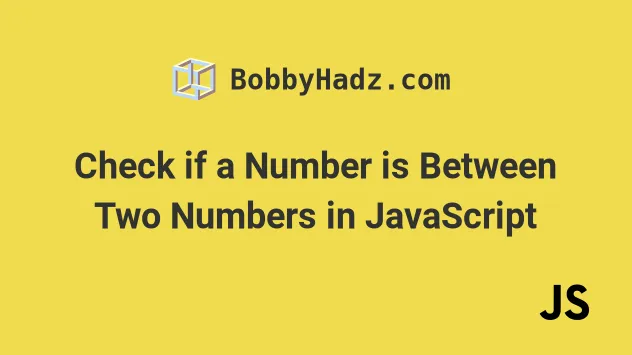## #Check if a Number is Between Two Numbers

To check if a number is between two numbers:

1. Check if the number is greater than the lower range.
2. Check if the number is less than the higher range.
3. If both conditions are met, the number is between the two numbers.
index.js
```Copied!```const num = 50;

const low = 30;
const high = 150;

if (num > low && num < high) {
// 👇️ this runs
console.log('✅ num is between the two numbers');
} else {
console.log('⛔️ num is NOT between the two numbers');
}
``````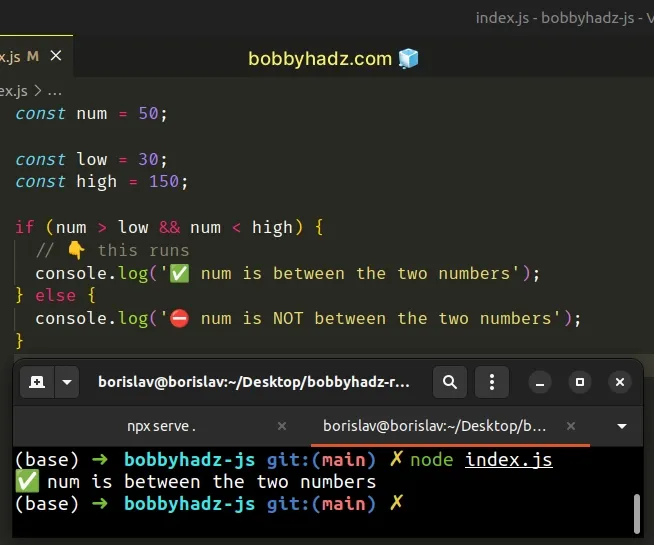If you aren't sure which of the two numbers is the lower number which is the greater number, use `Math.max()` and `Math.min()` instead.

index.js
```Copied!```// ✅ If you aren't sure which of the two numbers is low
// and which is high use Math.max() and Math.min()
const num = 50;

const low = 30;
const high = 150;

const max = Math.max(low, high);
console.log(max); // 👉️ 150

const min = Math.min(low, high);
console.log(min); // 👉️ 30

if (num > min && num < max) {
// 👇️ this runs
console.log('✅ num is between the two numbers');
} else {
console.log('⛔️ num is NOT between the two numbers');
}
``````

We used the logical AND (&&) operator to check if multiple conditions are true.

The `if` block only runs if the following two conditions are met:

• the number is greater than the lower value
• the number is less than the higher value
index.js
```Copied!```const num = 50;

const low = 30;
const high = 150;

if (num > low && num < high) {
// 👇️ this runs
console.log('✅ num is between the two numbers');
} else {
console.log('⛔️ num is NOT between the two numbers');
}
``````

If both conditions are met, the number is in the specified range, otherwise, it isn't.

The logical AND `&&` operator is evaluated from left to right. If the first condition in our `if` statement returns `false`, the operator short-circuits and doesn't evaluate the second condition.

## #Making the ranges inclusive

If you need to make the `low` and `high` ranges inclusive, use the `>=` and `<=` operators.

index.js
```Copied!```const num = 30;

const low = 30;
const high = 150;

if (num >= low && num <= high) {
// 👇️ this runs
console.log('✅ num is between the two numbers');
} else {
console.log('⛔️ num is NOT between the two numbers');
}
``````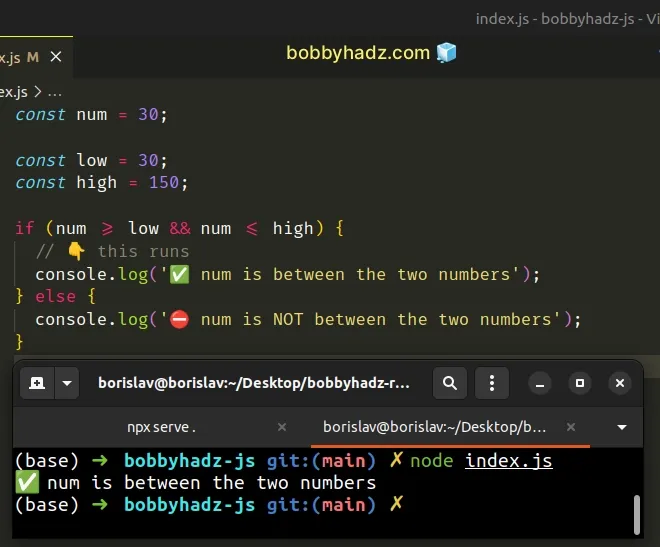The code sample checks if the number is greater than or equal to `low` and less than or equal to `high`.

## #Creating a reusable function

If you have to check if a number is between two numbers often, define a reusable function.

index.js
```Copied!```function numberInRange(num, low, high) {
if (num > low && num < high) {
return true;
}

return false;
}

console.log(numberInRange(5, 1, 10)); // 👉️ true

console.log(numberInRange(50, 1, 10)); // 👉️ false
``````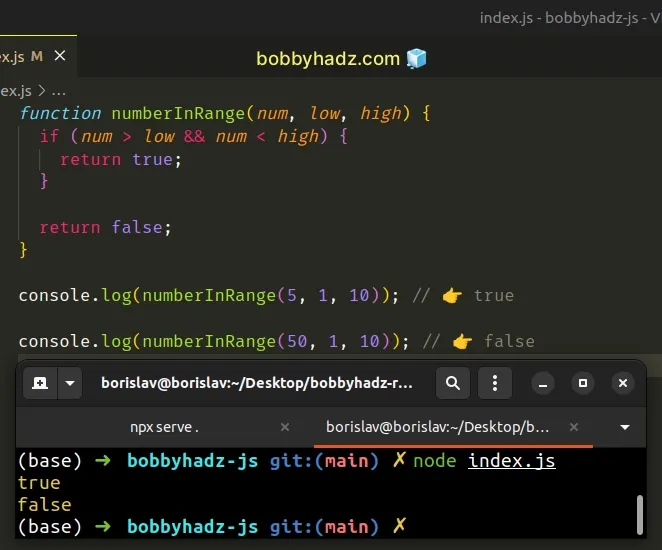The `numberInRange()` function takes 3 numbers as parameters and checks if the first number is between the other two numbers.

If you aren't sure which of the two numbers is `low` and which is `high`, use the `Math.max()` and `Math.min()` functions.

## #Check if a Number is between two numbers using `Math.max()` and `Math.min()`

This is a three-step process:

1. Use the `Math.max()` function to get the higher of the two numbers.
2. Use the `Math.min()` function to get the lower of the two numbers.
3. Check if the number is higher than the lower range and less than the higher range.
index.js
```Copied!```const num = 50;

const low = 30;
const high = 150;

const max = Math.max(low, high);
console.log(max); // 👉️ 150

const min = Math.min(low, high);
console.log(min); // 👉️ 30

if (num > min && num < max) {
// 👇️ this runs
console.log('✅ num is between the two numbers');
} else {
console.log('⛔️ num is NOT between the two numbers');
}
``````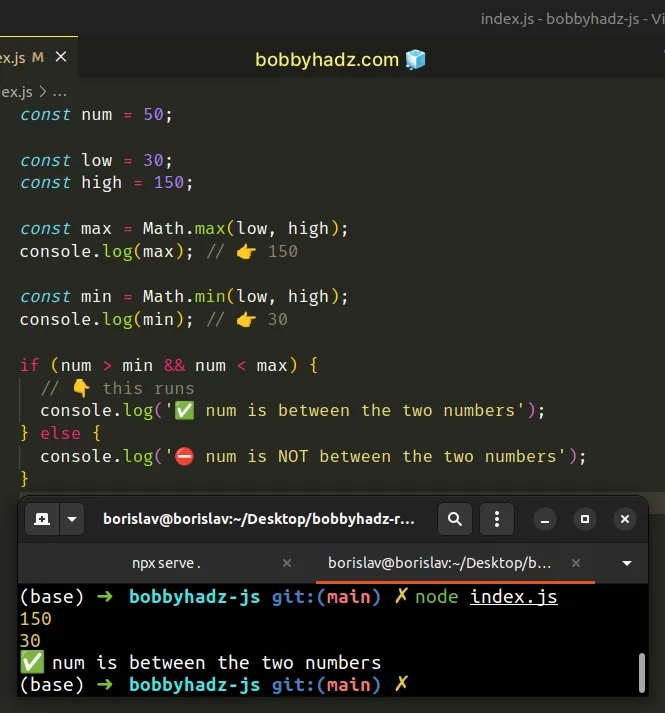This approach is useful when you don't know which of the two numbers is the higher number and which is the lower.

We used the `Math.max()` method to get the higher of the two numbers and the `Math.min()` method to get the lower number.

index.js
```Copied!```const first = 30;
const second = 150;

const max = Math.max(first, second);
console.log(max); // 👉️ 150

const min = Math.min(first, second);
console.log(min); // 👉️ 30
``````

Once we have the lower and higher ranges, we can use an `if` statement to check if the number is less than the higher range and greater than the lower range.

If both conditions are met, the number is between the two numbers.

## #Defining a reusable function

If you have to do this often, define a reusable function.

index.js
```Copied!```function numberInRange(num, first, second) {
const max = Math.max(first, second);
const min = Math.min(first, second);

if (num > min && num < max) {
return true;
}

return false;
}

console.log(numberInRange(5, 10, 50)); // 👉️ false

console.log(numberInRange(5, 1, 50)); // 👉️ true

console.log(numberInRange(5, 50, 1)); // 👉️ true
``````

The function takes a number and two other numbers that aren't bound to a specific order and returns `true` if the number is between the two numbers and `false` otherwise.

## #Including the lower and higher range

If you want to include the lower and higher range, change the conditions to check for less than or equal to and greater than or equal to.

index.js
```Copied!```const num = 30;

const low = 30;
const high = 150;

if (num >= low && num <= high) {
// 👇️ this runs
console.log('✅ num is between the two numbers');
} else {
console.log('⛔️ num is NOT between the two numbers');
}
``````

The `if` statement checks if the number is greater than or equal to the lower range and less than or equal to the higher range.

The logical AND `&&` operator is evaluated from left to right. If the first condition in our `if` statement returns `false`, the operator short-circuits and doesn't evaluate the second condition.

For example, in the following `if` statement, the `5 > 1` condition is never evaluated.

index.js
```Copied!```if (1 > 100 && 5 > 1) {
}
``````

We first check if `1 > 100` and get a value of `false`, so the `&&` operator short-circuits.

The `if` block is only run if both conditions are met and the number is in the specified range.

## #Check if a Number is between two numbers using the ternary operator

You can also use the ternary operator to check if a number is between two numbers.

index.js
```Copied!```const num = 50;

const low = 30;
const high = 150;

const inRange = num > low && num < high ? true : false;

if (inRange) {
// 👇️ this runs
console.log('The number is in the specified range');
} else {
console.log('The number is NOT in the specified range');
}
``````

The ternary operator is very similar to an `if/else` statement.

If the expression to the left of the question mark is truthy, the operator returns the value to the left of the colon, otherwise, the value to the right of the colon is returned.

You can imagine that the value before the colon is the `if` block and the value after the colon is the `else` block.

We first check if the `num` variable is greater than `low` and then check if it is lower than `high`.

If the condition is met, we return `true`, otherwise, `false` is returned.

## #Check if a Number is between two numbers using `lodash`

If you use the `lodash` library, you can also check if a number is in a certain range by using the lodash.inRange method.

index.js
```Copied!```import _ from 'lodash';

const num = 50;

const low = 30;
const high = 150;

console.log(_.inRange(num, low, high)); // 👉️ true

if (_.inRange(num, low, high)) {
// 👇️ this runs
console.log('The number is in the range');
} else {
console.log('The number is NOT in the range');
}
``````

If you need to install the `lodash` library, run the following command.

If you need to install `lodash`, run the following command.

shell
```Copied!```# 👇️ initialize a package.json file
npm init -y

npm install lodash
``````

The `lodash.inRange` method takes the following parameters:

1. `number` - the number to check.
2. `start` - the start of the range (inclusive).
3. `end` - the end of the range (up to, but not including).

The `lodash.inRange` method checks if a number is between `start` and up to, but not including `end`.

index.js
```Copied!```
console.log(_.inRange(50, 0, 100)); // 👉️ true

console.log(_.inRange(300, 0, 100)); // 👉️ false

console.log(_.inRange(0, -100, 50)); // 👉️ true
``````

If `end` is not specified, it is set to `start` and `start` is then set to `0`.

If `start` is greater than `end`, the parameters are swapped to support negative ranges.